###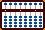Multifactorial Multiplication

The following is a very powerful technique for solving multiplication problems that contain two or more multipliers. When explaining the technique I'll use standard terminology. For example in the problem 1 x 2 x 3 = 6; the number 1 is the multiplicand, both 2 and 3 are the multipliers and 6 is the product.

When using the standard method to solve problems of multiplication on a soroban, product answers are routinely placed immediately to the right of the multiplicand. The standard method remains very efficient. But when a multiplication problem contains two or more multipliers the position of the unit number in a product moves with each operation. This can result in some confusion. This technique offers a very good solution.

Setting Numbers onto the Soroban

When solving problems using the standard Japanese method, the operator clears the multiplicand after each multiplication step. In this technique products are actually added to the multiplicand. In other words the multiplicand becomes a part of the product. As a result, when setting multipliers onto the soroban the value of each should be reduced by 1.

For example in 26 x 7 x 3 = 546, when setting numbers onto the soroban the multipliers 7 and 3 should each be reduced by 1 and placed onto the soroban as 6 and 2.

Example:  26 x 7 x 3 = 546

Step 1: Designate rod K to be the unit rod. Set the multiplicand 26 on rods JK. Set the first multiplier 6 (7-1) on rod E and the second multiplier 2 (3-1) on rod B. (Fig.1)(Fig.1) ```Step 1 A B C D E F G H I J K L M N O . . . .   .   0 2 0 0 6 0 0 0 0 2 6 0 0 0 0 Step 1 ```

Step 2:
Multiply 2 on rod J by the 6 on E and add the product 12 to rods IJ.

2a: Multiply 6 on on rod K by 6 on E. Add the product 36 to rods JK.

2b: Having finished with the 6 on E it can be cleared from the frame. This leaves the partial product of 182. (Fig.2)(Fig.2) ```Step 2 A B C D E F G H I J K L M N O . . . .   .   0 2 0 0 6 0 0 0 0 2 6 0 0 0 0 + 1 2 Step 2 0 2 0 0 6 0 0 0 1 4 6 0 0 0 0 + 3 6 Step 2a 0 2 0 0 6 0 0 0 1 8 2 0 0 0 0 (-6) Step 2b 0 2 0 0 0 0 0 0 1 8 2 0 0 0 0 ```

Step 3: Multiply 1 on rod I by 2 on rod B and add the product 02 to rods HI.

3a: Multiply 8 on rod J by 2 on B. Add the product 16 to rods IJ.

3b & the answer: Multiply 2 on rod K by 2 on B and add the product 04 to rods JK, leaving the answer 546 on rods IJK. (Fig.3)(Fig.3) ```Step 3 A B C D E F G H I J K L M N O . . . .   .   0 2 0 0 0 0 0 0 1 8 2 0 0 0 0 + 0 2 Step 3 0 2 0 0 0 0 0 0 3 8 2 0 0 0 0 + 1 6 Step 3a 0 2 0 0 0 0 0 0 5 4 2 0 0 0 0 + 0 4 Step 3b 0 2 0 0 0 0 0 0 5 4 6 0 0 0 0 ```

Determine the Unit Rod

With this technique, problems that involve multiplying mixed decimal and pure decimal numbers are easily solved:

• Designate a unit rod, set the problem and do the multiplication. Then for every decimal number in the multiplier(s) shift the unit rod one rod to the left.

Example:  2.6 x 0.017 x 1.4 = 0.06188

In this example notice the multipliers. The first multiplier 0.017 has three decimal numbers. The second multiplier 1.4 has one decimal number for a total of four decimal numbers. After solving the problem shift the unit rod four rods to the left.

Step 1: Choose rod I to be the unit rod. Set multiplicand 2.6 on rods IJ. Remembering that the multipliers are decimal fractions set 16 (17 -1) on rods DE and 13 (14 -1) on rods AB. (Fig.4)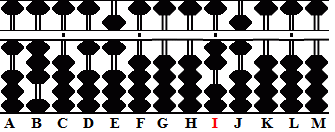(Fig.4) ```Step 1 A B C D E F G H I J K L M . . . .   1 3 0 1 6 0 0 0 2 6 0 0 0 Step 1 ```

Step 2: Multiply 2 on rod I by 1 on D and add the product 02 to rods GH.

2a: Multiply 2 on rod I by 6 on E and add the product 12 to rods HI.

2b: Multiply 6 on rod J by 1 on D and add the product 06 to rods HI.

2c: Multiply 6 on rod J by 6 on E and add the product 36 to rods IJ.

2d: Having finished with the multiplier 16 on rods DE, it can be cleared from the frame. This leaves the partial product of 442. (Fig.5)(Fig.5) ```Step 2 A B C D E F G H I J K L M . . . .   1 3 0 1 6 0 0 0 2 6 0 0 0 + 0 2 Step 2 + 1 2        Step 2a 1 3 0 1 6 0 0 3 4 6 0 0 0 + 0 6 Step 2b + 3 6        Step 2c 1 3 0 0 0 0 0 4 4 2 0 0 0```

Step 3: Multiply 4 on rod H by 1 on A. Add the product 04 to rods FG.

3a: Multiply 4 on rod H by 3 on B and add the product 12 to rods GH.

3b: Multiply 4 on rod I by 1 on A. Add the product 04 to rods GH.

3c: Multiply 4 on rod I by 3 on B and add the product 12 to rods HI.

3d: Multiply 2 on rod J by 1 on A. Add the product 02 to rods HI.

3e: Multiply 2 on rod J by 3 on B and add the product 06 to rods IJ leaving 6188.

Determine the unit rod and the final answer: Because the multipliers have a total of 4 decimal numbers count four rods to the left from rod I. Rod E is the new unit rod and the answer reads 0.06188. (Fig.6)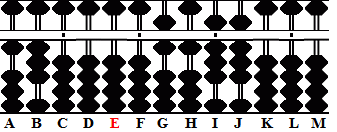(Fig.6) ```Step 3 A B C D E F G H I J K L M . . . .   1 3 0 0 0 0 0 4 4 2 0 0 0 + 0 4 Step 3 + 1 2        Step 3a 1 3 0 0 0 0 5 6 4 2 0 0 + 0 4 Step 3b + 1 2         Step 3c 1 3 0 0 0 0 6 1 6 2 0 0 0 + 0 2 Step 3d + 0 6       Step 3e 1 3 0 0 0 0 6 1 8 8 0 0 0```

A Simple 12x Multiplication Exercise

Here we'll take a slightly different turn. Let's use the multifactorial method again but instead of multiplying by two multipliers, we'll multiply by only one. Multiplying by 12 is quite a common operation. Using the multifactorial method, it's very quick and easy to solve problems that involve multiplying by 12.

Example 143 x 12 = 1716

Step 1: Designate rod K to be the unit rod. Set the multiplicand 143 on rods IJK. Set the multiplier 11 (12-1) on rods DE. (Fig.7)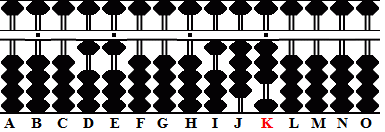Fig.7 ```Step 1 A B C D E F G H I J K L M N O . . . .   .   0 0 0 1 1 0 0 0 1 4 3 0 0 0 0 Step 1 ```

Step 2:
Multiply 1 on rod I by the 11 on DE and add the product 11 to rods HI.

2a: Multiply 4 on on rod J by 11 on DE. Add the product 44 to rods IJ.

2b & the answer: Multiply 3 on on rod K by 11 on DE. Add the product 33 to rods JK. This leaves the answer 1716. (Fig.8)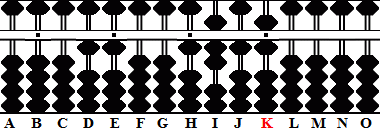Fig.8 ```Step 2 A B C D E F G H I J K L M N O . . . .   .   0 0 0 1 1 0 0 0 1 4 3 0 0 0 0 + 1 1 Step 2 0 0 0 1 1 0 0 1 2 4 3 0 0 0 0 + 4 4 Step 2a 0 0 0 1 1 0 0 1 6 8 3 0 0 0 0 + 3 3 Step 2b 0 0 0 1 1 0 0 1 7 1 6 0 0 0 0 ```

Real Life Situations

1) Let's say a customer buys 27 note books at a cost of \$1.25 each and has to pay a tax of 7%. In order to determine the total cost, the calculation would be 27 x 1.25 x 1.07 = 36.1125.

2) The area of a triangle can be found by multiplying one-half the base times the height. (Note: to find ½ of a number it can be multiplied by 0.5) If a triangle has a base length of 12 inches and a height of 7.5 inches, its area is 0.5 x 12 x 7.5 = 45 inches.

3) What is the volume and surface area of a cube having a side-length of 3.1 cm?
Its volume would be 3.1 × 3.1 × 3.1 = 29.791 cubic centimeters.
Its surface area would be 6 × 3.1 × 3.1 = 57.66 square centimeters.

 REFERENCES: Tejón, Fernando Kojima, Takashi The Japanese Abacus, Its use and Theory Tokyo: Charles E. Tuttle, 1954 Print Page (.pdf format,131kb)

June, 2005
Fernando Tejón
Totton Heffelfinger